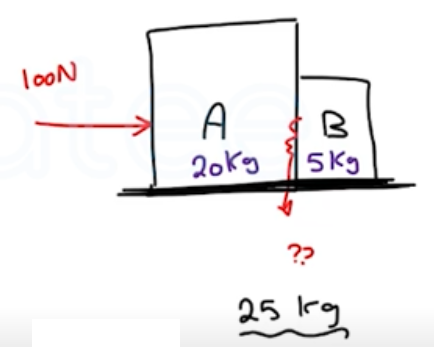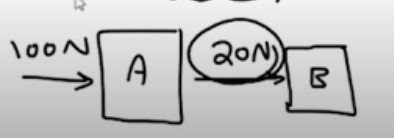Need Help?

Subscribe to Physics 1

###### \${selected_topic_name}
• Notes

$\begin{array}{l}{\text { At the surface of Jupiter's moon Io, the acceleration due }} \\ {\text { to gravity is } g=1.81 \mathrm{m} / \mathrm{s}^{2} \text { . A watermelon weighs } 44.0 \mathrm{N} \text { at the }} \\ {\text { surface of the earth. (a) What is the watermelon's mass on the }} \\ {\text { earth's surface? (b) What are its mass and weight on the surface }} \\ {\text { of Io? }}\end{array}$

$g=1.81 \mathrm{m/s}^{2}$

$g=9.8 \mathrm{m} / \mathrm{s}^{2}$

$\text { weighs }=44 \mathrm{N}$

$M_{w}. \ @ \ earth??$

$M_{w}, W_{w} @ \text { Jupiter } \text { ?? }$

$w=m g$

$m=\frac{w}{g}=\frac{44}{9.8}=4.49 \mathrm{kg}$

$w=m g$

$=4.49 *(1.81)=8.13 N$

$\begin{array}{l}{\text { Boxes } A \text { and } B \text { are in }} \\ {\text { contact on a horizontal, fric- }} \\ {\text { tionless surface, as shown in }} \\ {\text { Fig. E4.23. Box } A \text { has mass }} \\ {20.0 \mathrm{kg} \text { and box } B \text { has mass }} \\ {5.0 \mathrm{kg} \text { . A horizontal force of }} \\ {100 \mathrm{N} \text { is exerted on box } A .} \\ {\text { What is the magnitude of the }} \\ {\text { force that box } A \text { exerts on box } B \text { ? }}\end{array}$$\sum F=m a$

$a_{x}=\frac{F}{m}$

$=\frac{100}{25}=4 \mathrm{m/s}^{2}$

$\begin{array}{l}{a_{x}=4 m / s^{2}} \\ {\rightarrow}\end{array}$

$F=m a$

$=5 * 4=20 \mathrm{N}$$\begin{array}{l}{\text { A student with mass } 45 \text { kg jumps off a high diving board. }} \\ {\text { Using } 6.0 \times 10^{24} \text { kg for the mass of the earth, what is the acceler- }} \\ {\text { ation of the earth toward her as she accelerates toward the earth }} \\ {\text { with an acceleration of } 9.8 \mathrm{m} / \mathrm{s}^{2} ? \text { Assume that the net force on the }} \\ {\text { earth is the force of gravity she exerts on it. }}\end{array}$

$w=m g=(45 k g)(9.8)=441 N$

$\sum \vec{F}=m \vec{a}$

$441 \mathrm{N}=6 * 10^{24} \mathrm{kg} * a \rightarrow a=\frac{441}{6 * 10^{24}}=7.4*10^{-23} \mathrm{m/s}^2$